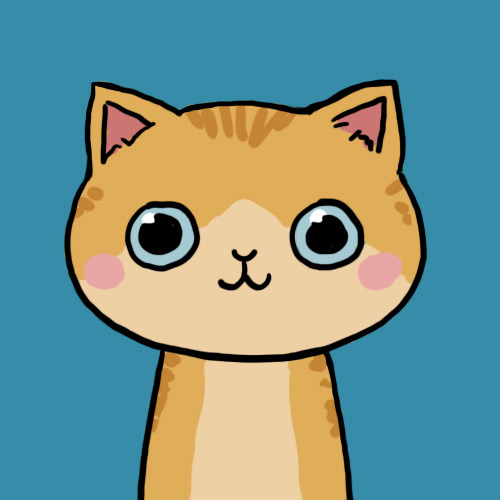Status:

Member Since: 15 Jul 2010 04:56pm

Last Seen: 16 Aug 2011 05:49pm

user id: 116633

1.posted a quote
September 11, 2010 2:39pm UTC
<3 <3 <3 ,3 <3 <3 <3<3<3<3<3
<3 <3 <3 <3 <3 <3
<3 <3 <3 <3 <3 <3<3<3<3
<3 <3 <3 <3 <3 <3
<3<3<3<3 <3 <3 <3 <3 <3<3<3<3<3

:)